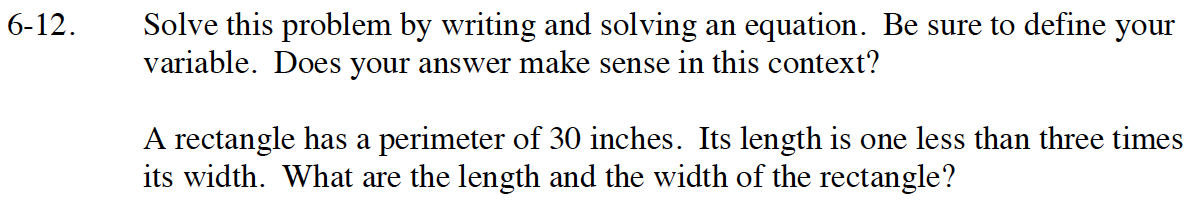Home > GB8I > Chapter cc46 > Lesson cc46.1.1 > Problem6-12

6-12.
1. Solve this problem by writing and solving an equation. Be sure to define your variable. Does your answer make sense in this context? Homework Help ✎

2. A rectangle has a perimeter of 30 inches. Its length is one less than three times its width. What are the length and the width of the rectangle?Set up two equations:
3W − 1 = L
2W + 2L = 30

Substitute (3W − 1) for L and solve the equation:
2W + 2 (3W − 1) = 30

Width is 4 inches and length is 11 inches.